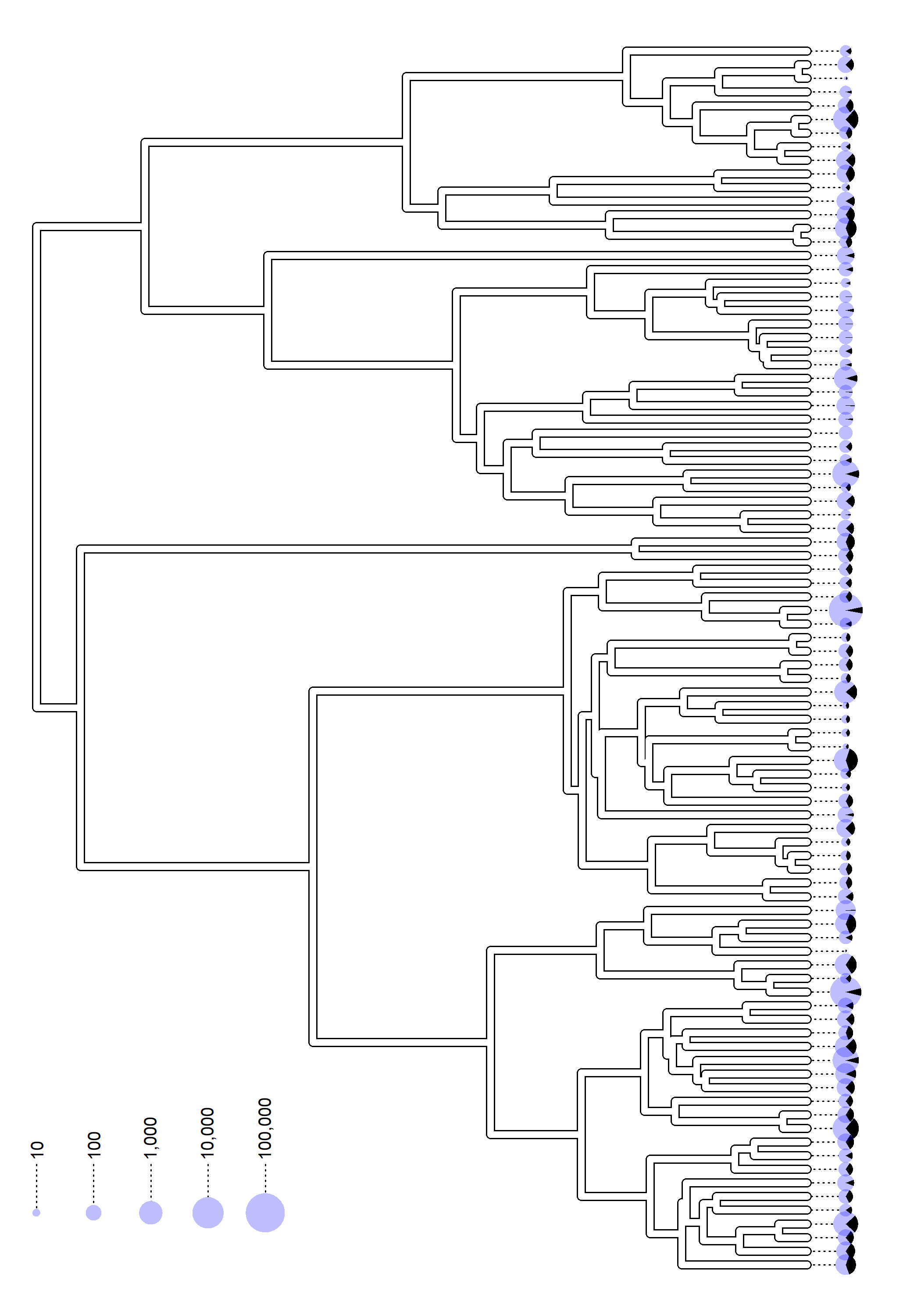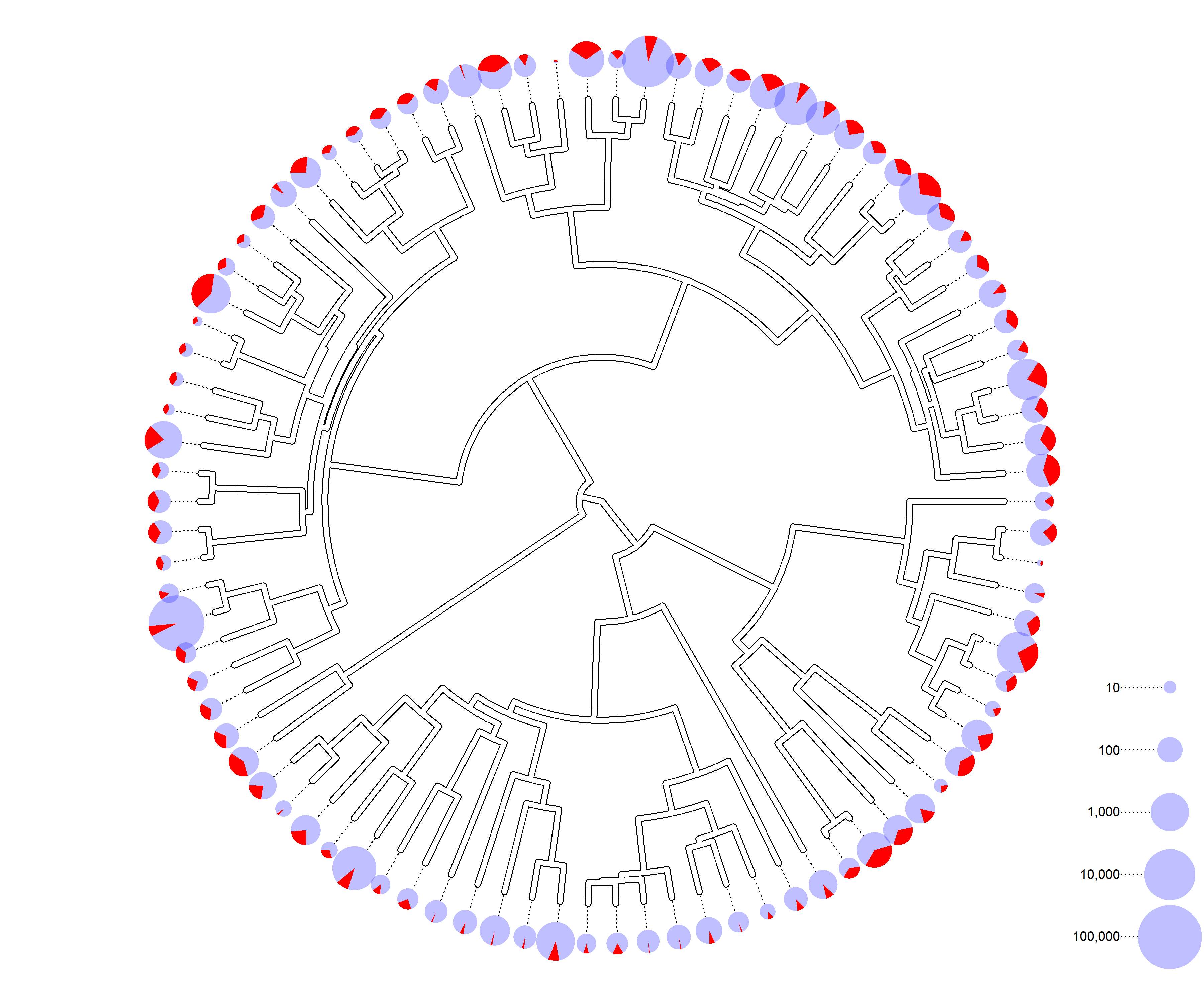## Thursday, July 21, 2022

### Graphing pie charts at the tips of a plotted tree scaled by sample size

As part of helping out a colleague with a project on disease prevalence across species, I developed some code to simultaneously illustrate relative frequency & sample size at the tips of the tree in R. Since this could be of interest to some other R phytools users, I thought I would share it here.

Unfortunately, aside from my colleague's data (which is part of ongoing project), I don't have any similar, relative frequency data to use to demo this visualization technique – so, I have to simulate it.

For my first example, I'll use a real primate phylogeny (from Kirk & Kay 2004 and used in my upcoming book with Luke Harmon); and simulated data generated on that tree. I'm going to simulate data that could conceivably look like disease prevalence – with observed relative frequencies between (more or less) 0 and 50%.

``````## load phytools & plotrix
library(phytools)
library(plotrix)
print(primate.tree,printlen=3)
``````
``````##
## Phylogenetic tree with 90 tips and 89 internal nodes.
##
## Tip labels:
##   Allenopithecus_nigroviridis, Cercopithecus_mitis, Cercopithecus_cephus, ...
##
## Rooted; includes branch lengths.
``````
``````## simulate p
p<-fastBM(primate.tree,bounds=c(0,0.4),sig2=0.001,a=0.25)
## simulate sample sizes
n<-setNames(ceiling(exp(rnorm(n=Ntip(primate.tree),
mean=log(100),sd=2))),primate.tree\$tip.label)+1
## simulate counts
counts<-round(p*n)
## simulate observed p
obs.p<-counts/n
``````

I'll start by plotting a right-facing square phylogram.

In this example I'll show a few different things.

First I'll “plot” my phylogeny without really plotting it (we talk about this a bit in Chapter 13 of the book). This allows me to open the graphical device and compute the x & y limits, as well as the coordinates of my plot, but without actually graphing the tree.

Next, to draw dotted lines extending from the tips of the tree to my plotting pie charts, I'll simply create a “temporary” `"phylo"` object (`tmp`), extend all the tip edges, and the plot the tree using dotted lines by setting `par(lty="dotted")`.

Then I plot my tree twice to create an outline effect, finally I plotting my floating pie charts twice – this is just so I can create a white background color above the lines of my tree before adding my pie charts with transparency on top of that. To make my prevalence slice align with the terminal edge of the tree, I simply split the prevalence in two and plotted slices `c(p/2,1-p,p/2)`.

Lastly, note that here I have sized the radii of my tip pie charts by the logarithm of the sample size for each species. I could've also done this on the original scale if desired (or sized the area by the sample size).

``````## plot tree without plotting
plotTree(primate.tree,ftype="off",plot=FALSE)
## get x & y limits for plotted tree
XLIM<-1.1*get("last_plot.phylo",envir=.PlotPhyloEnv)\$x.lim
YLIM<-get("last_plot.phylo",envir=.PlotPhyloEnv)\$y.lim
## copy tree into temporary phylo object & extend terminal edges
tmp<-primate.tree
tmp\$edge.length[which(primate.tree\$edge[,2]<=Ntip(primate.tree))]<-
tmp\$edge.length[which(primate.tree\$edge[,2]<=Ntip(primate.tree))]+
0.05*max(nodeHeights(primate.tree))
## plot tree using dotted lines, adding to open plot
par(lty="dotted",lend=0)
par(lty="solid")
## get coordinates of tips for pie charts
xx<-get("last_plot.phylo",envir=.PlotPhyloEnv)\$xx[1:Ntip(tmp)]
yy<-get("last_plot.phylo",envir=.PlotPhyloEnv)\$yy[1:Ntip(tmp)]
## add original tree to plot, overplotting to create outline effect
lwd=7,lend=0)
lwd=5,color="white",lend=0)
## normalize the sample sizes across species
ww<-diff(range(XLIM))
rr<-log(n)/max(log(n))*0.02*ww
par(fg="transparent")
for(i in 1:Ntip(primate.tree)){
floating.pie(xpos=xx[i],ypos=yy[i],
x=1,
col="white")
}
for(i in 1:Ntip(primate.tree)){
PP<-obs.p[primate.tree\$tip.label[i]]
floating.pie(xpos=xx[i],ypos=yy[i],
x=c(PP/2,1-PP,PP/2),
col=c("black",make.transparent("blue",0.25),"black"))
}
par(fg="black")
nn<-c(10,100,1000,10000,100000)
np<-length(nn)
pp<-log(nn)/max(log(n))*0.02*ww
xx<-seq(0,0.25,length.out=np)*diff(par()\$usr[1:2])
yy<-rep(0.05*diff(par()\$usr[3:4]),np)
for(i in 1:length(xx)){
lines(rep(xx[i],2),c(yy[i],yy[i]+
0.04*diff(par()\$usr[3:4])),
lty="dotted")
}
text(x=xx,y=yy+0.04*diff(par()\$usr[3:4]),
labels=prettyNum(nn,big.mark=",",scientific=FALSE),
par(fg="transparent")
for(i in 1:length(pp))
for(i in 1:length(pp))
col=make.transparent("blue",0.25))
````````````par(fg="black")
``````

Well that's pretty cool. Now let's try a fan style tree. Here it will be an extra challenge to orient our pie slices (as I'd like to do) along the terminal axis of each tip edge.

To do this I computed the start position of the pie slices as `atan(yy[i]/xx[i])` (for the ith tip), making appropriate adjustments for x < 0.

``````XLIM<-YLIM<-c(-1.15,1.15)*max(nodeHeights(primate.tree))
tmp<-primate.tree
tmp\$edge.length[which(primate.tree\$edge[,2]<=Ntip(primate.tree))]<-
tmp\$edge.length[which(primate.tree\$edge[,2]<=Ntip(primate.tree))]+
0.1*max(nodeHeights(primate.tree))
par(lty="dotted",lend=0)
plotTree(tmp,ftype="off",xlim=XLIM,ylim=YLIM,lwd=1,lend=0,
type="fan")
xx<-get("last_plot.phylo",envir=.PlotPhyloEnv)\$xx[1:Ntip(tmp)]
yy<-get("last_plot.phylo",envir=.PlotPhyloEnv)\$yy[1:Ntip(tmp)]
par(lty="solid")
lwd=7,lend=0,type="fan")
lwd=5,color="white",lend=0,type="fan")
ww<-diff(range(XLIM))
rr<-log(n)/max(log(n))*0.03*ww
par(fg="transparent")
for(i in 1:length(xx)){
floating.pie(xpos=xx[i],ypos=yy[i],
x=1,
edges=400,
col="white")
}
for(i in 1:length(xx)){
PP<-obs.p[primate.tree\$tip.label[i]]
startpos<-atan(yy[i]/xx[i])
if(xx[i]<0) startpos<-pi+startpos
floating.pie(xpos=xx[i],ypos=yy[i],
x=c(PP/2,1-PP,PP/2),
edges=400,
col=c("red",make.transparent("blue",0.25),"red"),
startpos=startpos)
}
par(fg="black")
nn<-c(10,100,1000,10000,100000)
np<-length(nn)
pp<-log(nn)/max(log(n))*0.03*ww
xx<-rep(par()\$usr-max(pp),np)
yy<-par()\$usr+2*max(pp)+seq(0.25,0,length.out=np)*
diff(par()\$usr[3:4])
for(i in 1:length(xx)){
lines(c(xx[i],xx[i]-0.05*diff(par()\$usr[3:4])),
rep(yy[i],2),lty="dotted")
}
text(x=xx-0.05*diff(par()\$usr[3:4]),y=yy,
labels=prettyNum(nn,big.mark=",",scientific=FALSE),
par(fg="transparent")
for(i in 1:length(pp))
for(i in 1:length(pp))
col=make.transparent("blue",0.25))
````````````par(fg="black")
``````

That's pretty cool, right?